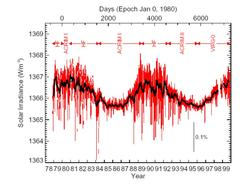MERLOT Biology Portal Visit Main MERLOTMerlot Biology Pedagogic CollectionTime Series Analysis is a fascinating field and its full treatment can easily exceed the scope of an introductory geoscience course. However, trend analysis and an introductory exposure to spectral analysis may both be appropriate for your introductory college courses. The linear trend or slope of a graph is often a useful value to estimate by hand from a series of data. learn more here Least Squares Linear Regression is often introduced in introductory geoscience courses to varying degrees of mathematical sophistication. learn more here Fourier transforms of time series or spatial data are used throughout the geosciences. Consider providing your introductory geoscience students with some familiarity of this essential analysis tool. learn more here